# Partial derivative online calculator

Partial derivative concept is only valid to the multivariable functions. Examine two variable function z=f( x,y). Partial derivative by variables x and y are denoted as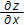and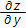correspondingly. The partial derivativesandby themselfs are also the two variable functions: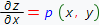and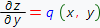, so their partial derivatives can also be found:Derivatives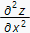andare the second order partial derivatives of the function z by the variables x and y correspondingly. Derivativesandare called mixed derivatives of the function z by the variables x, y and y, x correspondingly. If the function z and their mixed derivativesandare defined at some neighborhood of a point M(x0,y0) and continuous at that point, then the following equality is valid:Similary, one can introduce the higher order derivatives, for instancemeans that we should differentiate the function z two times by the variable x and three times by the variable y, so:Sometimes, in order to denote partial derivatives of some function z=f(x,y) notations fx'(x,y) and fy'(x,y) are used. Subscript index is used to indicate the differentiation variable. Using this approach one can denote mixed derivatives: fxy''(x,y) and fyx''(x,y) and also the second and higher order derivatives: fxx''(x,y) and fxxy'''(x,y) accordingly. Following notations are equivalent:, however, usually digit 1 is absent. To denote partial derivatives in our online calculator, we use symbols:. Example of step by step solution can be found here.

## Choose expression input type:

Expression input type:

## Choose differentiation variables and the orders by these variables:

Differentiation variable

Derivative order .

Differentiation variable

Derivative order .